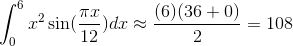AP Calculus AB : Trapezoidal sums

Example Questions

Example Question #31 : Trapezoidal Sums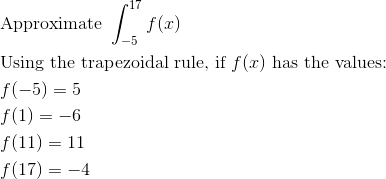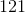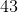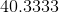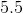Explanation: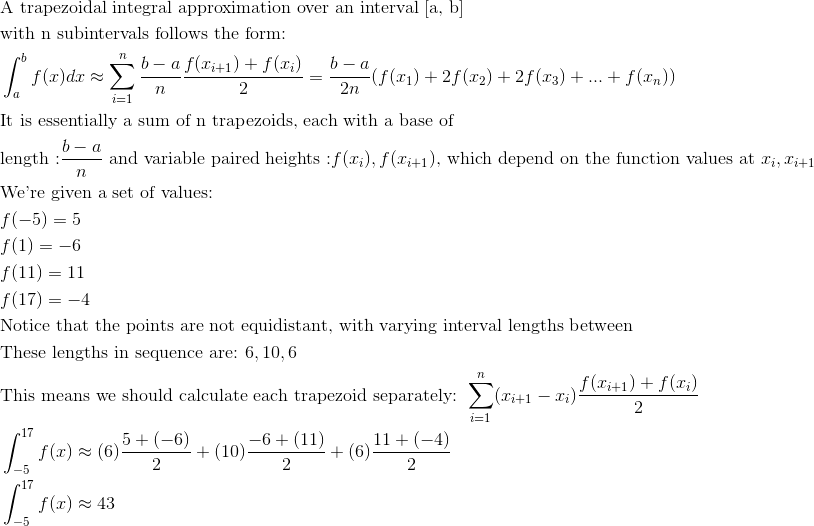Example Question #32 : Trapezoidal Sums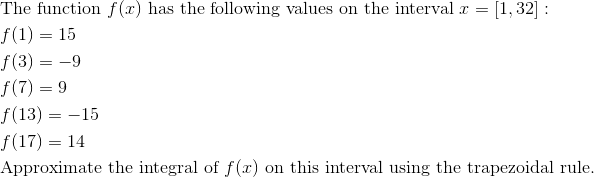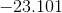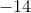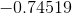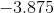Explanation: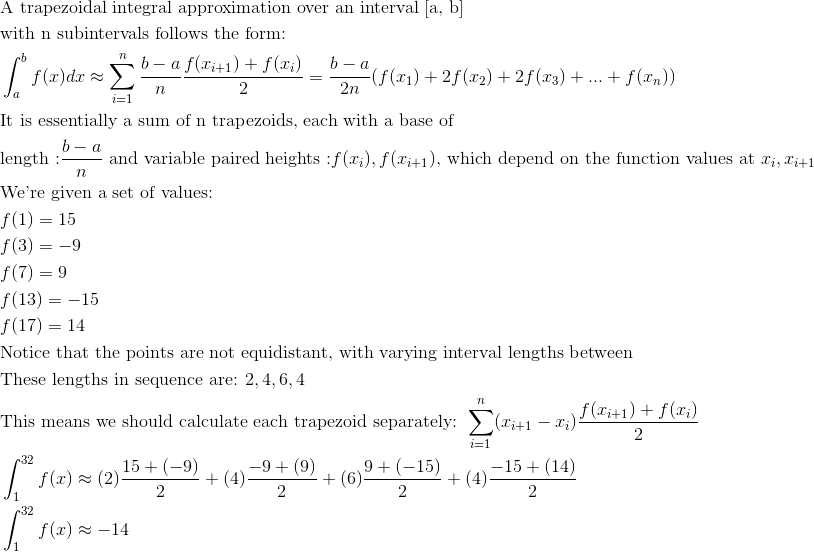Example Question #33 : Trapezoidal Sums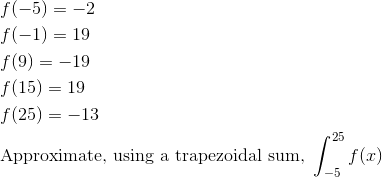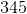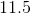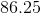Explanation: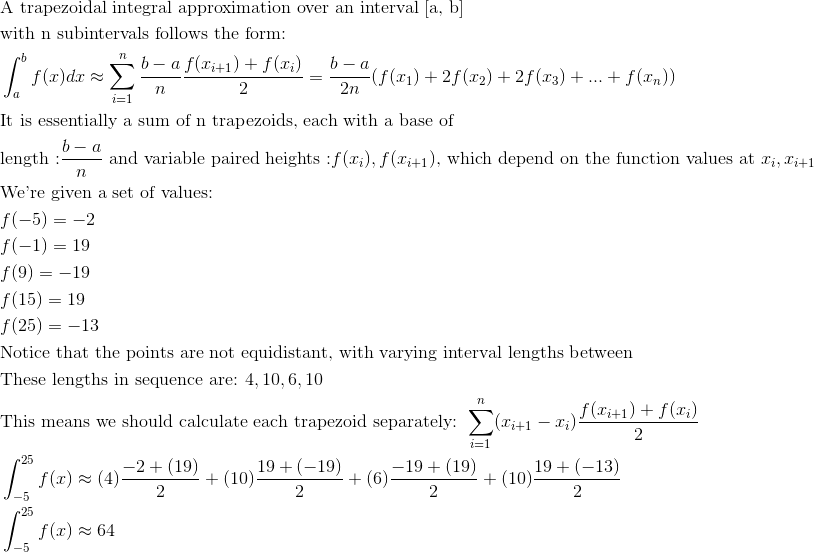Example Question #34 : Trapezoidal Sums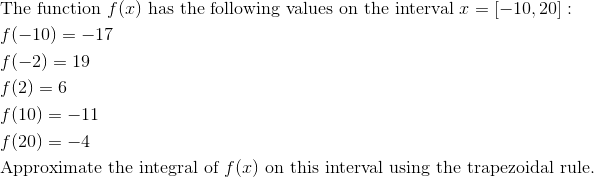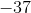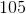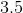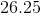Explanation: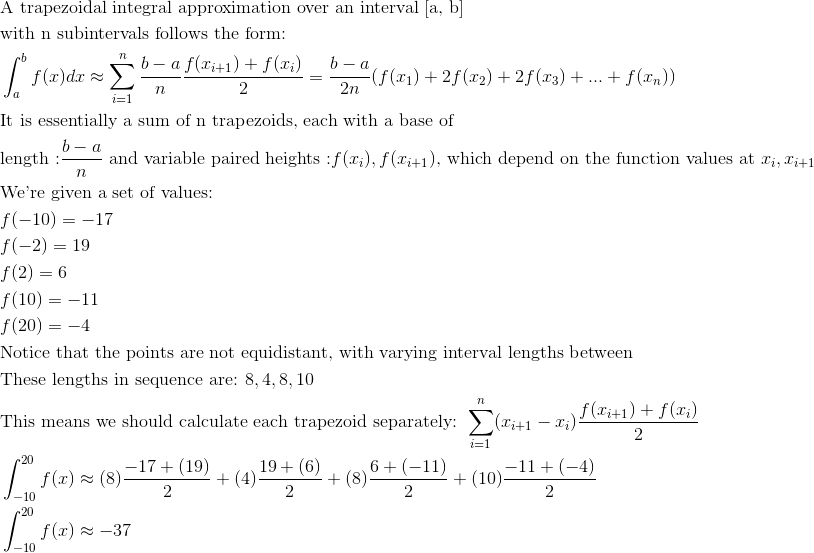Example Question #35 : Trapezoidal Sums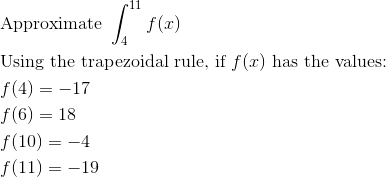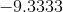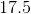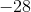Explanation: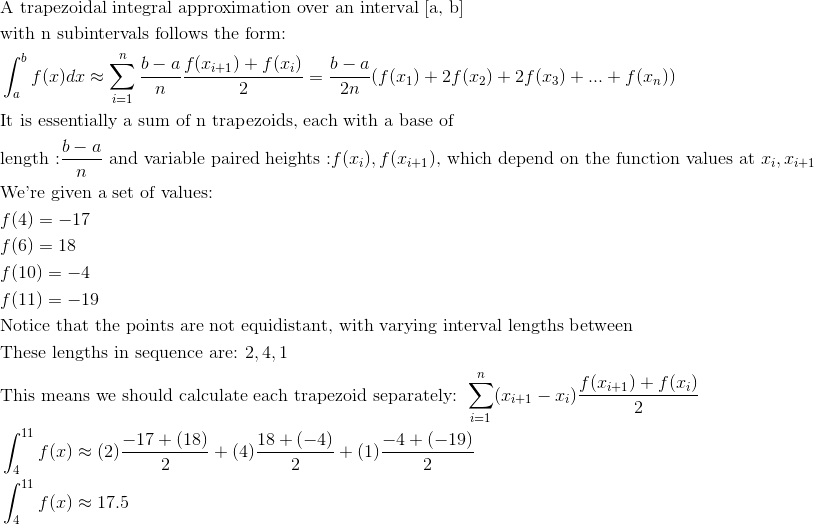Example Question #36 : Trapezoidal Sums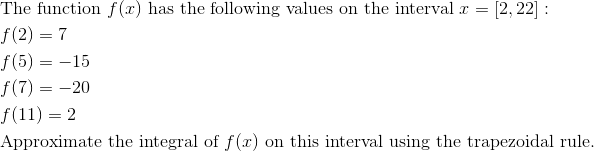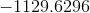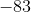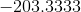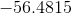Explanation: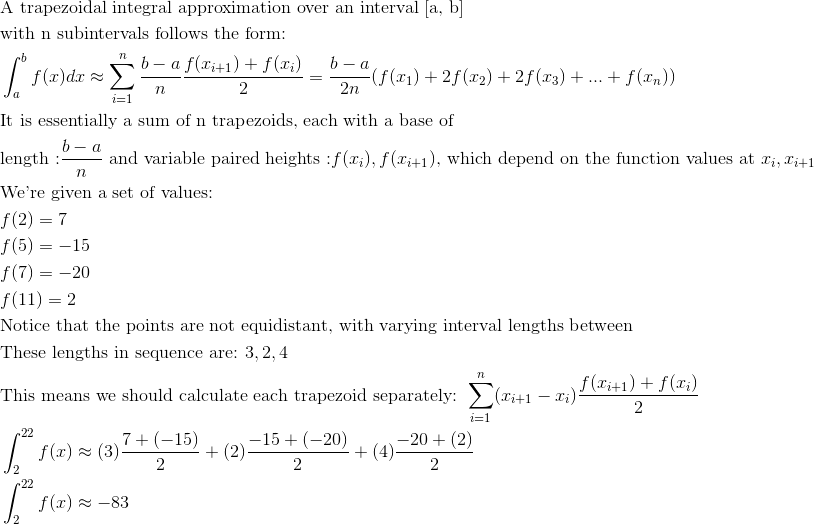Example Question #37 : Trapezoidal Sums

Use the trapezoidal rule to approximate the following integral: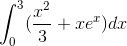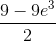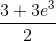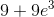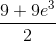Explanation:

The trapezoidal rule for approximating a definite integral is given by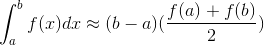Using the above formula to approximate our integral, we get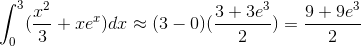Example Question #38 : Trapezoidal Sums

Solve using the trapezoidal approximation: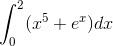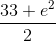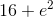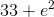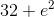Explanation:

The trapezoidal approximation of a definite integral is given by the following: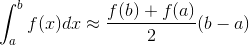Using this approximation for our integral, we get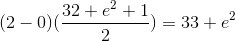Example Question #39 : Trapezoidal Sums

Solve the integral using the trapezoidal approximation: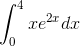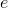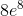Explanation:

To approximate the definite integral using the trapezoidal rule, we use the following approximation: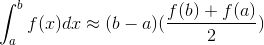For our integral, we get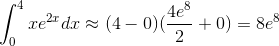Example Question #40 : Trapezoidal Sums

Approximate the integral using the trapezoidal rule: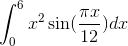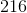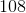Explanation:

To approximate the definite integral using the trapezoidal rule, we use the following approximation:For our integral, we get